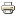Separating equilibrium in quasi-linear signaling gamesWorking paper
Author/s:
Rudolf Müller, Dries Vermeulen
Issue number:
26.2014
Series:
Maastricht University, Graduate School of Business and Economics (GSBE) Research Memorandum
Year:
2014
Using a network approach we provide a characterization of a separating equilibrium for standard signaling games where the senders payoff function is quasi-linear. Given a strategy of the sender, we construct a network where the node set and the length between two nodes are the set of the senders type and the difference of signaling costs, respectively. Construction of a separating equilibrium is then equivalent to constructing the length between two nodes in the network under the condition that the response of the receiver is a node potential.We show that, when the set of the senders type is finite, the collection of separating signaling functions forms a lower bounded lattice. We describe an algorithm to compute separating equilibrium strategies. When the set of the senders type is a real interval, shortest path lengths are antisymmetric and a node potential is unique up to a constant. A strategy of the sender in a separating equilibrium is characterized by some differential equation with a unique solution.Our results can be readily applied to a broad range of economic situations, such as the standard job market signaling model of Spence a model not captured by earlier papers and principal-agent models with production.
Developed by Paolo Gittoi